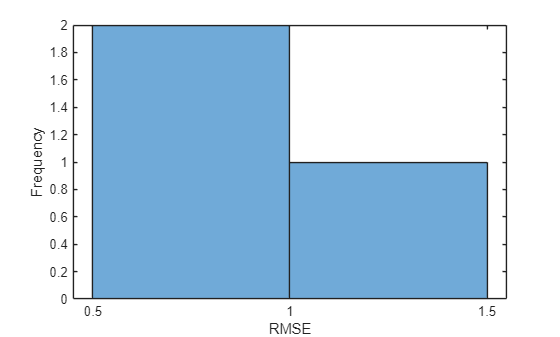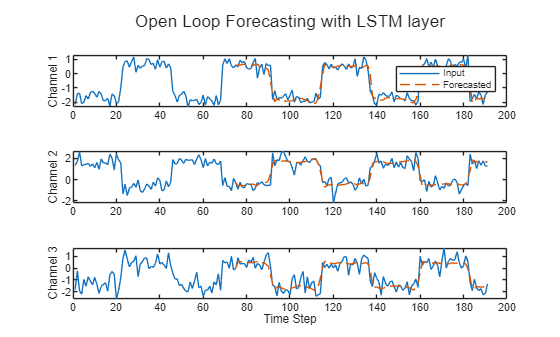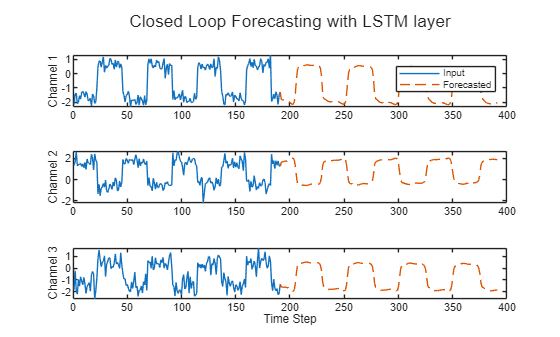# Run Sequence Forecasting on FPGA by Using Deep Learning HDL Toolbox™

This example shows how to create, compile, and deploy a long short-term memory (LSTM) network trained on waveform data by using the Deep Learning HDL Toolbox™ Support Package for Xilinx FPGA and SoC. Use the deployed network to predict future values by using open-loop and closed-loop forecasting. Use MATLAB® to retrieve the prediction results from the target device.

### Waveform Data Network

The network attached to this example was trained using the Time Series Forecasting Using Deep Learning. This example uses the `WaveformData.mat` data set, which contains 2000 synthetically generated waveforms of varying lengths with three channels. This example uses a trained LSTM network to forecast future values of the waveforms given the values from the previous time steps using both closed loop and open loop forecasting.

### Prerequisites

• Xilinx® Zynq® Ultrascale+™ ZCU102 SoC development kit

• Deep Learning HDL Toolbox™ Support Package for Xilinx FPGA and SoC

• Deep Learning Toolbox™

• Deep Learning HDL Toolbox™

To load the LSTM network enter:

`load WaveformForcastingNet`

Use the `analyzeNetwork` function to obtain information about the network layers. the function returns a graphical representation of the network that contains detailed parameter information for every layer in the network.

`analyzeNetwork(net)`### Define FPGA Board Interface

Define the target FPGA board programming interface by using the `dlhdl.Target` object. Specify that the interface is for a Xilinx board with an Ethernet interface.

To create the target object, enter:

`hTarget = dlhdl.Target('Xilinx','Interface','Ethernet');`

To use the JTAG interface, install Xilinx™ Vivado™ Design Suite 2020.2. To set the Xilinx Vivado toolpath, enter:

```hdlsetuptoolpath('ToolName', 'Xilinx Vivado', 'ToolPath', 'C:\Xilinx\Vivado\2020.2\bin\vivado.bat'); hTarget = dlhdl.Target('Xilinx','Interface','JTAG'); ```

### Prepare Network for Deployment

Prepare the network for deployment by creating a `dlhdl.Workflow` object. Specify the network and the bitstream name. Ensure that the bitstream name matches the data type and the FPGA board. In this example the target FPGA board is the Xilinx ZCU102 SOC board. The bitstream uses a single data type.

`hW = dlhdl.Workflow('network', net, 'Bitstream', 'zcu102_lstm_single','Target',hTarget);`

Tu run the example on the Xilinx ZC706 board, enter:

```hW = dlhdl.Workflow('Network', snet, 'Bitstream', 'zc706_lstm_single','Target',hTarget); ```

### Compile the LSTM Network

Run the `compile` method of the `dlhdl.Workflow` object to compile the network and generate the instructions, weights, and biases for deployment. The total number of frames exceeds the default value of 30. Set the `InputFrameNumberLimit` name-value argument to `1000` to run predictions in chunks of 1000 frames to prevent timeouts.

`dn = compile(hW,'InputFrameNumberLimit',1000)`
```### Compiling network for Deep Learning FPGA prototyping ... ### Targeting FPGA bitstream zcu102_lstm_single. ### The network includes the following layers: 1 'sequenceinput' Sequence Input Sequence input with 3 dimensions (SW Layer) 2 'lstm' LSTM LSTM with 128 hidden units (HW Layer) 3 'fc' Fully Connected 3 fully connected layer (HW Layer) 4 'regressionoutput' Regression Output mean-squared-error with response 'Response' (SW Layer) ### Notice: The layer 'sequenceinput' with type 'nnet.cnn.layer.ImageInputLayer' is implemented in software. ### Notice: The layer 'regressionoutput' with type 'nnet.cnn.layer.RegressionOutputLayer' is implemented in software. ### Compiling layer group: lstm.wi ... ### Compiling layer group: lstm.wi ... complete. ### Compiling layer group: lstm.wo ... ### Compiling layer group: lstm.wo ... complete. ### Compiling layer group: lstm.wg ... ### Compiling layer group: lstm.wg ... complete. ### Compiling layer group: lstm.wf ... ### Compiling layer group: lstm.wf ... complete. ### Compiling layer group: fc ... ### Compiling layer group: fc ... complete. ### Allocating external memory buffers: offset_name offset_address allocated_space _______________________ ______________ ________________ "InputDataOffset" "0x00000000" "4.0 MB" "OutputResultOffset" "0x00400000" "4.0 MB" "SchedulerDataOffset" "0x00800000" "4.0 MB" "SystemBufferOffset" "0x00c00000" "20.0 MB" "InstructionDataOffset" "0x02000000" "4.0 MB" "FCWeightDataOffset" "0x02400000" "4.0 MB" "EndOffset" "0x02800000" "Total: 40.0 MB" ### Network compilation complete. ```
```dn = struct with fields: weights: [1×1 struct] instructions: [1×1 struct] registers: [1×1 struct] syncInstructions: [1×1 struct] constantData: {} ```

To deploy the network on the Xilinx ZCU102 SoC hardware, run the `deploy` function of the `dlhdl.Workflow` object. This function uses the output of the `compile` function to program the FPGA board by using the programming file. It also downloads the network weights and biases. The `deploy` function starts programming the FPGA device and displays progress messages, and the required time to deploy the network.

` deploy(hW)`

### Test Network

Prepare the test data for prediction. Normalize the test data using the statistics calculated from the training data. To forecast the values of future time steps of a sequence, specify the targets as the test sequences with values shifted by one time step. In other words, at each time step of the input sequence, the LSTM network learns to predict the value of the next time step. The predictors as the test sequences without the final time step.

```load Waveformdata numChannels = size(data{1},1); numObservations = numel(data); idxTrain = 1:floor(0.9*numObservations); idxTest = floor(0.9*numObservations)+1:numObservations; dataTrain = data(idxTrain); dataTest = data(idxTest); for n = 1:numel(dataTrain) X = dataTrain{n}; XTrain{n} = X(:,1:end-1); TTrain{n} = X(:,2:end); end muX = mean(cat(2,XTrain{:}),2); sigmaX = std(cat(2,XTrain{:}),0,2); muT = mean(cat(2,TTrain{:}),2); sigmaT = std(cat(2,TTrain{:}),0,2); for n = 1:size(dataTest,1) X = dataTest{n}; XTest{n} = (X(:,1:end-1) - muX) ./ sigmaX; TTest{n} = (X(:,2:end) - muT) ./ sigmaT; end```

Make predictions using the test data.

`YTest = hW.predict(XTest{1});`
```### Resetting network state. ### Finished writing input activations. ### Running a sequence of length 115. ```

To evaluate the accuracy, calculate the root mean squared error (RMSE) between the predictions and the target for each test sequence.

```for i = 1:size(YTest,1) rmse(i) = sqrt(mean((YTest(i) - TTest{1}(i)).^2,"all")); end```

Visualize the errors in a histogram. Lower values indicate greater accuracy.

```figure histogram(rmse) xlabel("RMSE") ylabel("Frequency")```Calculate the mean RMSE over all test observations.

`mean(rmse)`
```ans = single 0.8385 ```

### Forecast Future Time Steps

To forecast the values of multiple future time steps, when given an input time series or sequence, use the `predictAndUpdateState` function. This function predicts time steps one at a time and updates the network state at each prediction. For each prediction, use the previous prediction as the input to the function.

Visualize one of the test sequences in a plot.

```idx = 2; X = XTest{idx}; T = TTest{idx}; figure stackedplot(X',DisplayLabels="Channel " + (1:numChannels)) xlabel("Time Step") title("Test Observation " + idx)```#### Open-Loop Forecasting

Open-loop forecasting predicts the next time step in a sequence using only the input data. When making predictions for subsequent time steps, you collect the true values form your data source and use those as input. For example, suppose that you want to predict the value for time step $t$ of a sequence by using data collected in time steps 1 through $t-1$. To make predictions for time step $t+1$, wait until you record the true value for time step $t$ and use that value as input to make the next prediction. Use open-loop forecasting when you have true values to provide to the network before making the next prediction.

Initialize the network state by resetting the state using the `resetState` function, then make an initial prediction using the first few time steps of the input data. Update the network state by using the first 75 time steps of the input data.

```resetState(hW) offset = 75; [~,~] = hW.predictAndUpdateState(X(:,1:offset)); ```
```### Resetting network state. ### Finished writing input activations. ### Running a sequence of length 75. ```

To forecast further predictions, loop over time steps and update the network state by using the `predictAndUpdateState` function. Forecast values for the remaining time steps of the test observation by looping over the time steps of the input data and using them as input to the network. The first prediction is the value that corresponds to the time step `offset + 1`.

```numTimeSteps = size(X,2); numPredictionTimeSteps = numTimeSteps - offset; Y = zeros(numChannels,numPredictionTimeSteps); for t = 1:numPredictionTimeSteps Xt = X(:,offset+t); Y(:,t) = predictAndUpdateState(hW,Xt); end```

Compare the predictions with the target values.

```figure t = tiledlayout(numChannels,1); title(t,"Open Loop Forecasting") for i = 1:numChannels nexttile plot(T(i,:)) hold on plot(offset:numTimeSteps,[T(i,offset) Y(i,:)],'--') ylabel("Channel " + i) end xlabel("Time Step") nexttile(1) legend(["Input" "Forecasted"])```#### Closed-Loop Forecasting

Closed-loop forecasting predicts subsequent time steps in a sequence by using the previous predictions as input. In this case, the model does not require the true values to make the prediction. For example, suppose that you want to predict the value for time steps $t$ through $t+k$ of the sequence by using data collected in time steps 1 through $t-1$. To make predictions for time step $i$, use the predicted value for time step $i-1$ as input. Use closed-loop forecasting to forecast multiple subsequent time steps or when you do not have true values to provide to the network before making the next prediction.

Initialize the network state by resetting the state using the `resetState` function, then make an initial prediction, `Z, `using the first few time steps of the input data. Update the network state by using the first 75 time steps of the input data.

```resetState(hW) offset = size(X,2); [Z, ~] = predictAndUpdateState(hW,X);```
```### Resetting network state. ### Finished writing input activations. ### Running a sequence of length 191. ```

To forecast further predictions, loop over time steps and update the network state by using the `predictAndUpdateState` function. Forecast the next 200 time steps by iteratively passing the previously predicted value to the network. Because the network does not require the input data to make any further predictions, you can specify any number of time steps to forecast.

```numPredictionTimeSteps = 200; Xt = Z(:,end); Y = zeros(numChannels,numPredictionTimeSteps); for t = 1:numPredictionTimeSteps [Y(:,t),~] = predictAndUpdateState(hW,Xt); Xt = Y(:,t); end```

Visualize the forecasted values in a plot.

```numTimeSteps = offset + numPredictionTimeSteps; figure t = tiledlayout(numChannels,1); title(t,"Closed Loop Forecasting") for i = 1:numChannels nexttile plot(T(i,1:offset)) hold on plot(offset:numTimeSteps,[T(i,offset) Y(i,:)],'--') ylabel("Channel " + i) end xlabel("Time Step") nexttile(1) legend(["Input" "Forecasted"])```Closed-loop forecasting allows you to forecast an arbitrary number of time steps, but can be less accurate when compared to open-loop forecasting because the network does not have access to the true values during the forecasting process.